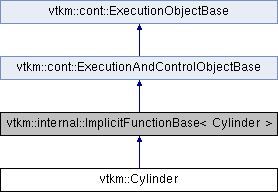VTK-m  2.0
vtkm::Cylinder Class Reference

Implicit function for a cylinder. More...

`#include <ImplicitFunction.h>`

Inheritance diagram for vtkm::Cylinder:## Public Member Functions

VTKM_EXEC_CONT Cylinder ()
Construct cylinder radius of 0.5; centered at origin with axis along y coordinate axis. More...

VTKM_EXEC_CONT Cylinder (const Vector &axis, Scalar radius)

VTKM_EXEC_CONT Cylinder (const Vector &center, const Vector &axis, Scalar radius)

VTKM_CONT void SetCenter (const Vector &center)

VTKM_CONT void SetAxis (const Vector &axis)

VTKM_EXEC_CONT Scalar Value (const Vector &point) const

VTKM_EXEC_CONT Vector Gradient (const Vector &point) const

Vector Center

Vector Axis

## Detailed Description

Implicit function for a cylinder.

`Cylinder` computes the implicit function and function gradient for a cylinder using F(r)=r^2-Radius^2. By default the Cylinder is centered at the origin and the axis of rotation is along the y-axis. You can redefine the center and axis of rotation by setting the Center and Axis data members.

Note that the cylinder is infinite in extent.

## ◆ Cylinder() [1/3]

 VTKM_EXEC_CONT vtkm::Cylinder::Cylinder ( )
inline

Construct cylinder radius of 0.5; centered at origin with axis along y coordinate axis.

## ◆ Cylinder() [2/3]

 VTKM_EXEC_CONT vtkm::Cylinder::Cylinder ( const Vector & axis, Scalar radius )
inline

## ◆ Cylinder() [3/3]

 VTKM_EXEC_CONT vtkm::Cylinder::Cylinder ( const Vector & center, const Vector & axis, Scalar radius )
inline

## Member Function Documentation

 VTKM_EXEC_CONT Vector vtkm::Cylinder::Gradient ( const Vector & point ) const
inline

## ◆ SetAxis()

 VTKM_CONT void vtkm::Cylinder::SetAxis ( const Vector & axis )
inline

## ◆ SetCenter()

 VTKM_CONT void vtkm::Cylinder::SetCenter ( const Vector & center )
inline

inline

## ◆ Value()

 VTKM_EXEC_CONT Scalar vtkm::Cylinder::Value ( const Vector & point ) const
inline

## ◆ Axis

 Vector vtkm::Cylinder::Axis
private

## ◆ Center

 Vector vtkm::Cylinder::Center
private